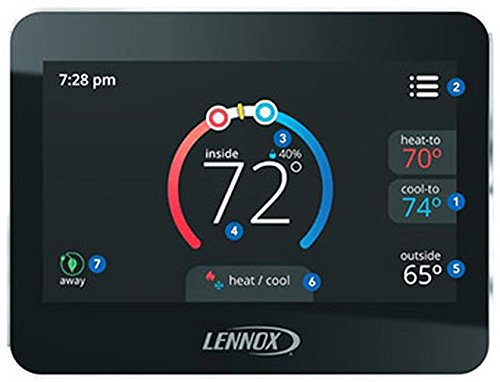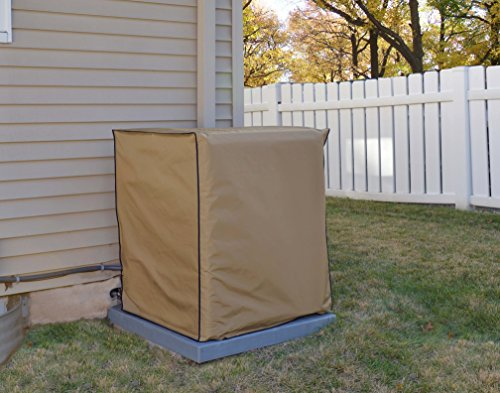# How Big Is A Lennox Air Conditioner?

When choosing appliances for your home, the size of these machines is always something to be considered. A Lennox air conditioning unit is no exception. This company has earned notoriety and consumers' trust for creating quality, dependable units, but how big can you expect them to be? We've done the research to give you an answer.

Lennox air conditioners have a cooling tonnage of 1.5 tons to 5 tons. The smallest of these units is 100-200 pounds in weight. The largest is up to 350 pounds.

The width of the largest of Lennox air conditioners is 35.5 inches, while the width of the smallest is 24.25 inches. The height of the largest units is 47 inches, while the height of the smallest is 27 inches.

Now we've given you a general idea of how large or small you can expect your unit to be, but there's a lot of area in between. You may want to know the sizing of the specific unit you plan to purchase, or you may have additional questions. Keep reading to learn more.## Lennox Air Conditioner Sizes

Lennox has many models of air conditioning units, and each is unique in its capabilities and sizing. Here, we'll discuss each model and give you their specifications.

### Lennox XC25

The Lennox XC25 has an impressively high SEER [Seasonal Energy Efficiency Ratio] rating of 26. This powerful air conditioning unit is produced with air cooling tonnages of 2, 3, 4, and 5. The weight and dimensions of each unit are as follows:

• The 2 ton XC25 is 303 pounds; dimensions are 35.5" wide and 47" tall.
• The 3 ton XC25 is 305 pounds; dimensions are 35.5" wide and 47" tall.
• The 4 ton XC25 is 329 pounds; dimensions are 35.5" wide and 47" tall.
• The 5 ton XC25 is 331 pounds; dimensions are 35.5" wide and 47" tall.Check out this Lennox smart thermostat on Amazon.

### Lennox XC21

The Lennox XC21 has a SEER rating of 21, which qualifies this model as being highly efficient. These units are produced with 2, 3, 4, and 5 cooling tonnage capabilities. The weight and dimensions of each of these units are as follows:

• The 2 ton XC21 is 314 pounds; dimensions are 35.5" wide and 47" tall.
• The 3 ton XC21 is 331 pounds; dimensions are 35.5" wide and 47" tall.
• The 4 ton XC21 is 337 pounds; dimensions are 35.5" wide and 47" tall.
• The 5 ton XC21 is 357 pounds; dimensions are 35.5" wide and 47" tall.

### Lennox SL 18XC1

The Lennox SL18XC1 model boasts a SEER rating of 18. This model can be purchased in several cooling tonnage capabilities, including 2, 2.5, 3, 3.5, 4, and 5 tons. Below is a list of the weight and dimensions of these units:

• The 2 ton SL18XC1 is 263 pounds; dimensions are 35.5" wide and 41" tall.
• The 2.5 ton SL18XC1 is 285 pounds; dimensions are 35.5" wide and 41" tall.
• The 3 ton SL18XC1 is 286 pounds; dimensions are 35.5" wide and 41" tall.
• The 3.5 ton SL18XC1 is 311 pounds; dimensions are 35.5" wide and 41" tall.
• The 4 ton SL18XC1 is 344 pounds; dimensions are 35.5" wide and 47" tall.
• The 5 ton SL18XC1 is 349 pounds; dimensions are 35.5" wide and 47" tall.

### Lennox XC20

The Lennox XC20 model has been attributed with a SEER rating of 20. This unit is produced in 2, 3, 4, and 5 cooling tons. The weight and dimensions of these air conditioning units are as follows:

• The 2 ton XC20 is 243 pounds; dimensions are 35.5" wide and 39" tall.
• The 3 ton XC20 is 241 pounds; dimensions are 35.5" wide and 39" tall.
• The 4 ton XC20 is 287 pounds; dimensions are 35.5" wide and 45" tall.
• The 5 ton XC20 is 321 pounds; dimensions are 35.5" wide and 45" tall.

### Lennox XC16

The Lennox XC16 has a 16 SEER rating. It can be found in 2, 3, 4, and 5 cooling tonnage capabilities. The specifications of this model are as follows:

• The 2 ton XC16 is 236 pounds; dimensions are 30.5" wide and 45" tall.
• The 3 ton XC16 is 243 pounds; dimensions are 30.5" wide and 31" tall.
• The 4 ton XC16 is 323 pounds; dimensions are 30.5" wide and 39" tall.
• The 5 ton XC16 is 332 pounds; dimensions are 30.5" wide and 45" tall.

### Lennox EL16XC1

The Lennox EL16XC1 also has a 16 SEER rating. It can be purchased in 1.5, 2, 2.5, 3, 3.5, 4, and 5 cooling tons. Below are the weight and dimensions of each of these units:

• The 1.5 ton EL16XC1 is 165 pounds; dimensions are 27" wide and 31" tall.
• The 2 ton EL16XC1 is 185 pounds; dimensions are 31" wide and 35" tall.
• The 2.5 ton EL16XC1 is 213 pounds; dimensions are 30.5" wide and 39" tall.
• The 3 ton EL16XC1 is 240 pounds; dimensions are 30.5" wide and 31" tall.
• The 3.5 ton EL16XC1 is 270 pounds; dimensions are 30.5" wide and 39" tall.
• The 4 ton EL16XC1 is 290 pounds; dimensions are 35.5" wide and 35" tall.
• The 5 ton EL16XC1 is 290 pounds; dimensions are 35.5" wide and 45" tall.

### Lennox XC13

The Lennox XC13 has a SEER rating of 13. It can be found in 1.5, 2, 2.5, 3, 3.5, 4, and 5 cooling tons. The weight and dimensions of each of these units are as follows:

• The 1.5 ton XC13 is 151 pounds; dimensions are 27" wide and 27" tall.
• The 2 ton XC13 is 152 pounds; dimensions are 27" wide and 27" tall.
• The 2.5 ton XC13 is 165 pounds; dimensions are 27" wide and 31" tall.
• The 3 ton XC13 is 170 pounds; dimensions are 27" wide and 31" tall.
• The 3.5 ton XC13 is 232 pounds; dimensions are 30.5" wide and 39" tall.
• The 4 ton XC13 is 225 pounds; dimensions are 30.5" wide and 35" tall.
• The 5 ton XC13 is 247 pounds; dimensions are 31" wide and 35" tall.

### Lennox 16ACX

The Lennox 16ACX has a 16 SEER rating. It is produced with 2, 3, 4, and 5 cooling tonnage capabilities. The weight and dimensions of these products are listed below:

• The 2 ton 16ACX is 212 pounds; dimensions are 28.25" wide and 43.25" tall.
• The 3 ton 16ACX is 230 pounds; dimensions are 28.25" wide and 29.25" tall.
• The 4 ton 16ACX is 262 pounds; dimensions are 28.25" wide and 37.25" tall.
• The 5 ton 16ACX is 332 pounds; dimensions are 32.25" wide and 43.25" tall.

### Lennox ML14XC1

The Lennox ML14XC1 has a 14 SEER rating. It can be purchased in 1.5, 2, 2.5, 3, 3.5, 4, and 5 tons. The weight and dimensions of these units are as follows:

• The 1.5 ton ML14XC1 is 135 pounds; dimensions are 24.25" wide and 29.25" tall.
• The 2 ton ML14XC1 is 165 pounds; dimensions are 28.25" wide and 29.25" tall.
• The 2.5 ton ML14XC1 is 170 pounds; dimensions are 28.25" wide and 37.25" tall.
• The 3 ton ML14XC1 is 190 pounds; dimensions are 28.25" wide and 37.25" tall.
• The 3.5 ton ML14XC1 is 210 pounds; dimensions are 28.25" wide and 37.25" tall.
• The 4 ton ML14XC1 is 235 pounds; dimensions are 32.25" wide and 33.25" tall.
• The 5 ton ML14XC1 is 235 pounds; dimensions are 32.25" wide and 43.25" tall.Take a look at this air conditioner cover compatible with Lennox ML14XC1.

### Lennox 13ACX

The Lennox 13ACX has a 13 SEER rating. It is available in 1.5, 2, 2.5, 3, 3.5, 4, and 5 cooling tons. The weight and dimensions of this model are listed below:

• The 1.5 ton 13ACX is 130 pounds; dimensions are 24.25" wide and 25.25" tall.
• The 2 ton 13ACX is 135 pounds; dimensions are 24.25" wide and 25.25" tall.
• The 2.5 ton 13ACX is 133 pounds; dimensions are 24.25" wide and 29.25" tall.
• The 3 ton 13ACX is 135 pounds; dimensions are 24.25" wide and 29.25" tall.
• The 3.5 ton 13ACX is 178 pounds; dimensions are 28.25" wide and 29.25" tall.
• The 4 ton 13ACX is 216 pounds; dimensions are 28.25" wide and 33.25" tall.
• The 5 ton 13ACX is 236 pounds; dimensions are 28.25" wide and 29.25" tall.Now that you know the specifications of Lennox air conditioning models, you may have some other questions about your air conditioning unit and home. We'll answer some of the most commonly asked here.

### How Do You Tell What Size Your AC Unit Is?

You can determine the size of your Lennox air conditioner by locating the identification plate on the outside of the unit, reading the model number, and searching for the model number on the Lennox website. You will find the model's specifications, including the cooling tonnage, weight, and dimensions.

### What Size AC Unit Do You Need For 1500 Sq Feet?

A 2.5-ton air conditioning unit is the correct size for a 1500 square feet home. It will adequately cool the house so that it is comfortable but will not be too powerful for the size of the home.

## Related QuestionsMaybe you have more questions about Lennox air conditioning units. We'll answer some of the most frequently asked questions.

### What Is The Standard Size Of An Air Conditioner?

Air conditioner units are sized based on their cooling tonnage. While there are no one-size-fits-all air conditioning units, there is a standard of 1 ton per 600 square feet. For example, if a home is 1800 square feet, it will require an air conditioning unit with 3 tons.

### How Do You Know The Tonnage Of A Lennox Air Conditioner?You can find the tonnage of a Lennox air conditioner by locating the identification plate on the outside of the unit, reading the model number, and plugging this model number into the search bar on the Lennox website. You will find all of the specifications for the model there, including the cooling tonnage.

## In ConclusionLennox is a highly trusted brand for HVAC products, but even so, you have to consider the size of these units to decide on the correct product for your home. We've given you the specifications of every model, from the smallest to the largest. We hope that this information will be helpful to you in your search.